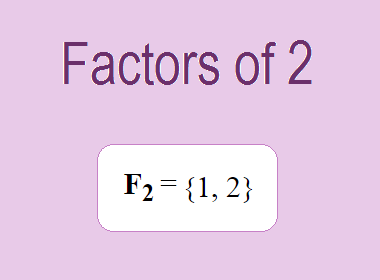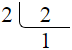# Factors of 2The factors of 2 are 1 and 2 i.e. F2 = {1, 2}. The factors of 2 are all the numbers that can divide 2 without leaving a remainder. 2 is a prime number, so it is divisible by 1 and 2 only.

We can check if these numbers are factors of 2 by dividing 2 by each of them. If the result is a whole number, then the number is a factor of 2. Let's do this for each of the numbers listed above:

·        1 is a factor of 2 because 2 divided by 1 is 2.

·        2 is a factor of 2 because 2 divided by 2 is 1.

## Properties of the Factors of 2

The factors of 2 have some interesting properties. One of the properties is that the sum of the factors of 2 is equal to 3. We can see this by adding all the factors of 2 together:

1 + 2 = 3

Another property of the factors of 2 is that the only prime factor of 2 is 2 itself.

********************

********************

## Applications of the Factors of 2

The factors of 2 have several applications in mathematics. One of the applications is in finding the highest common factor (HCF) of two or more numbers. The HCF is the largest factor that two or more numbers have in common. For example, to find the HCF of 2 and 10, we need to find the factors of both numbers and identify the largest factor they have in common. The factors of 2 are 1, and 2. The factors of 10 are 1, 2, 5, and 10. The largest factor that they have in common is 2. Therefore, the HCF of 2 and 10 is 2.

Another application of the factors of 2 is in prime factorization. Prime factorization is the process of expressing a number as the product of its prime factors. The prime factor of 2 is 2 since it is only the prime number that can divide 2 without leaving a remainder. Therefore, we can express 2 as:

2 = 2

We can do prime factorization by division method as given below,2 = 2

Since 2 is a prime number, there is no factor tree of 2.

## Conclusion

The factors of 2 are the numbers that can divide 2 without leaving a remainder. The factors of 2 are 1, and 2. The factors of 2 have some interesting properties, such as having a sum of 3. The factors of 2 have several applications in mathematics, such as finding the highest common factor and prime factorization.